Courses

# Turbulent Flow Civil Engineering (CE) Notes | EduRev

## Civil Engineering (CE) : Turbulent Flow Civil Engineering (CE) Notes | EduRev

The document Turbulent Flow Civil Engineering (CE) Notes | EduRev is a part of the Civil Engineering (CE) Course Civil Engineering SSC JE (Technical).
All you need of Civil Engineering (CE) at this link: Civil Engineering (CE)

Chapter 11 Turbulent Flow

•  Velocity distribution is relatively uniform and velocity profile is much flatter than the corresponding laminar  flow parabola for the same mean velocity, as shown below :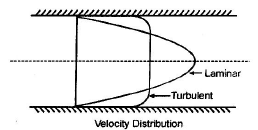• Shear stress in turbulent flow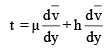where,
μ = dynamic coefficient of viscosity (fluid characteristic)
h = eddy viscosity coefficient (flow characteristic)

•  eddy viscosity come into picture due to turbulence effect
•  Hydro-Dynamically Smooth And Rough Pipes
•  If the average height of irregularities (k) is greater than the thickness of laminar sublayer (d'), then the  boundary is called hydrodynamically Rough.
• If the average height of irregularities (k) is less than the thickness of laminar sublayer (d'), then the  boundary is called hydrodynamically smooth.
• On the basis of NIKURADSE's EXPERIMENT the boundary is classified as :

Hydrodynamically smooth : (k/d) < 0.25

Boundary in transition :0.25 < (k/d)< 6.0

Hydrodynamically Rough :(k/d) > 6.0

• (R/K) is known as specific roughness. where ‘k’ is average height of roughness and ‘R’ is radius of the pipe.

• Velocity Distribution For Turbulent Flow in Pipes

(a) Prandtl’s universal velocity distribution equation :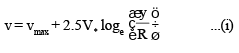where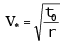shear or friction velocity.
y = distance from pipe wall

•  The above equation is valid for both smooth and rough pipe boundaries.

(b) Karman - Prandtl Velocity distribution equation :
(i) Hydro Dynamically Smooth pipe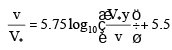(ii) Hydro Dynamically Rough pipe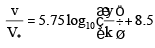where
V* = shear velocity
y = distance from pipe wall
k = average height of roughness
v = kinematic viscosity.
(c) Velocity distribution in terms of mean velocity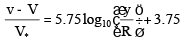The above equation is for both rough and smooth pipes.

• Friction Factor

(a) Friction factor ‘f’ for laminar flow :
f = (64/Re) where Re = Reynolds number
(b) Friction factor ‘f’ for transition flow :
There exists no specific relationship between f and Re for transition flow in pipes.
(c) Friction factor (f) for turbulent flow in smooth pipes :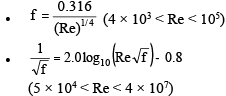(d) Friction factor (f) for turbulent flow in rough pipes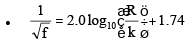This equation shows that for rough pipes friction factor depends only on R/K (Relative smoothness) and not on Reynolds number (Re)

Offer running on EduRev: Apply code STAYHOME200 to get INR 200 off on our premium plan EduRev Infinity!

## Civil Engineering SSC JE (Technical)

109 docs|50 tests

,

,

,

,

,

,

,

,

,

,

,

,

,

,

,

,

,

,

,

,

,

;